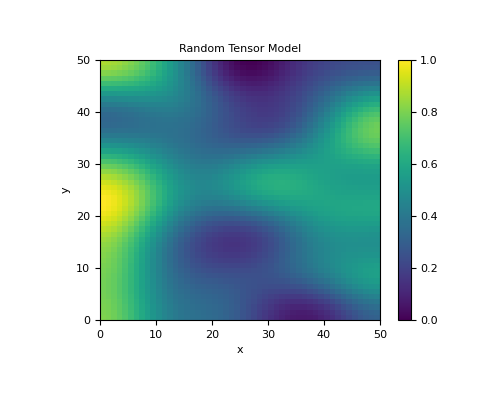# discretize.utils.random_model¶

discretize.utils.random_model(shape, seed=None, anisotropy=None, its=100, bounds=None)[source]

Create random tensor model.

Creates a random tensor model by convolving a kernel function with a uniformly distributed model. The user specifies the number of cells along the x, (y and z) directions with the input argument shape and the function outputs a tensor model with the same shape. Afterwards, the user may use the `mkvc()` function to convert the tensor to a vector which can be plotting on a corresponding tensor mesh.

Parameters
shape(`dim`) `tuple` `of` `int`

shape of the model.

seed`int`, `optional`

pick which model to produce, prints the seed if you don’t choose

anisotropy`numpy.ndarray`, `optional`

this is the kernel that is convolved with the model

its`int`, `optional`

number of smoothing iterations

bounds`list`, `optional`

Lower and upper bounds on the model. Has the form [lower_bound, upper_bound].

Returns
`numpy.ndarray`

A random generated model whose shape was specified by the input parameter shape

Examples

Here, we generate a random model for a 2D tensor mesh and plot.

```>>> from discretize import TensorMesh
>>> from discretize.utils import random_model, mkvc
>>> import matplotlib as mpl
>>> import matplotlib.pyplot as plt
```
```>>> h = [(1., 50)]
>>> vmin, vmax = 0., 1.
>>> mesh = TensorMesh([h, h])
```
```>>> model = random_model(mesh.shape_cells, seed=4, bounds=[vmin, vmax])
```
```>>> fig = plt.figure(figsize=(5, 4))
>>> ax = plt.subplot(111)
>>> im, = mesh.plot_image(model, grid=False, ax=ax, clim=[vmin, vmax])
>>> cbar = plt.colorbar(im)
>>> ax.set_title('Random Tensor Model')
>>> plt.show()
```